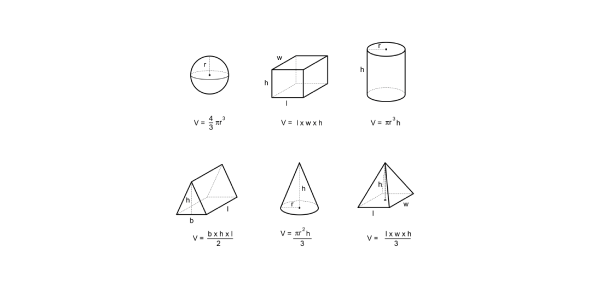# Geometry Perimeter, Area/Surface Area &volume

Approved & Edited by ProProfs Editorial Team
At ProProfs Quizzes, our dedicated in-house team of experts takes pride in their work. With a sharp eye for detail, they meticulously review each quiz. This ensures that every quiz, taken by over 100 million users, meets our standards of accuracy, clarity, and engagement.
| Written by Hpelto
H
Hpelto
Community Contributor
Quizzes Created: 1 | Total Attempts: 73
Questions: 6 | Attempts: 73Settings• 1.

### The formula for perimeter is

• A.

Length times width

• B.

Length times width times height

• C.

Length plus width plus length plus width

• D.

Length plus width

C. Length plus width plus length plus width
Explanation
The correct answer is "length plus width plus length plus width" because the formula for calculating the perimeter of a rectangle is to add up all the sides. In a rectangle, opposite sides are equal in length, so we add the length twice and the width twice to get the total perimeter.

Rate this question:

• 2.

### I need to figure out the perimeter when I want to purchase a rug for my bedroom floor.

• A.

True

• B.

False

B. False
Explanation
The statement is false because the perimeter is the measurement around the outside of a shape, such as a room or a rug. When purchasing a rug for a bedroom floor, the area of the floor would be more relevant than the perimeter, as the area would determine the size of the rug needed to cover the floor.

Rate this question:

• 3.

### What is volume

the amount of space something takes up
Explanation
Volume is a measurement that refers to the amount of space occupied by an object or substance. It quantifies the three-dimensional extent of an object and is typically measured in cubic units. In this context, the correct answer defines volume as the amount of space something takes up, which accurately captures the concept of volume.

Rate this question:

• 4.

### I have a possible perimeter of  _________ when I have an area of 24.

• A.

28

• B.

50

• C.

23

A. 28
B. 50
C. 23
Explanation
The possible perimeters that can be obtained when the area is 24 are 28, 50, and 23.

Rate this question:

• 5.

### I can't meaure the surface area of a kitchen floor

• A.

True

• B.

False

A. True
Explanation
The statement implies that the person is unable to measure the surface area of a kitchen floor. This suggests that they lack the necessary tools or skills to accurately determine the area. Therefore, the answer "True" indicates that it is not possible for the person to measure the surface area of a kitchen floor.

Rate this question:

• 6.

### I need to figure surface area for

• A.

How much tissue will fit in a tissue box

• B.

Wrapping a present

• C.

Table cloth

• D.

New necklaceBack to top